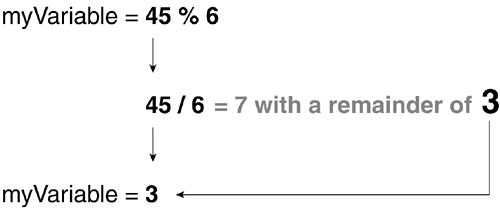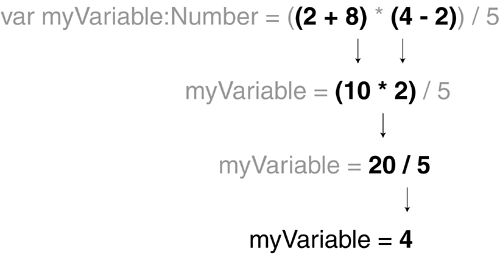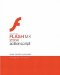# Operators

Operators are the marks within an expression that control the way in which the expression's values are evaluated. The operators you use will depend on the ways in which you need to manipulate values.

NOTE

In this section we review both arithmetic and string operators. For information about logical and comparison operators, see Lesson 8, "Using Conditional Logic."

#### Arithmetic Operators

Even if you're not very familiar with ActionScript, you'll recognize most of the arithmetic operators. These operators are used in expressions to manipulate numeric values.

• Addition operator (+). Adds two numeric values together. For example, var totalCost:Number = productPrice + tax adds the two variables to arrive at a final result.

• Increment operator (++). A shorthand method for adding 1 to a value. For example, ++myAge increases the value of the myAge variable by 1 the equivalent of myAge = myAge + 1;

• Subtraction operator ( ). This operator subtracts two values and can be used in the same way as the addition operator. For example, var moneyInWallet = paycheck - moneySpent subtracts one value from another to return a new number.

• Decrement operator (--). This operator reduces the value of a variable by 1. For example, --bottlesOfBeerOnTheWall takes a bottle of beer from the wall.

• Multiplication operator (*). This operator multiplies one numeric value by another. For example, in var hoursPerWeek:Number = 24 * 7 the number of hours per week is the product of these two numbers being multiplied together.

• Division operator (/). Divides one numeric value by another. For example: var hourlyRate:Number = payCheck / hoursBilled divides the value of hoursBilled into the value of payCheck.

• Modulo operator (%). Divides the value on the left by the value on the right and returns the value of the remainder. For example, in 4 % 2 the result would be 0 because 4 can be evenly divided by 2; hence there is no remainder. In 7 % 3, the result is 1 because 3 divides into 7 twice with a remainder of 1. Here's an illustration of how it works:#### String Operators

Unlike numbers, which can be manipulated using several different operators, strings can be manipulated by only one operator the concatenation operator (they can also be manipulated using various methods of the String class, however). Although other operators work with strings (namely assignment operators and comparison operators), they cannot be used to directly manipulate a string. (For more on comparison operators, see Lesson 8, "Using Conditional Logic.")

• Concatenation operator (+). Concatenation means to link or join two or more things exactly what this operator does with strings of text in ActionScript. The concatenation operator which uses the same symbol as the addition operator joins two text strings to create a single string. For example:

` var birthDayMessage:String = "You are " + age + " years old."; `

If age has a value of 26, then the plus symbol joins the three parts of the message together to form "You are 26 years old."

#### Precedence

Expressions can often include several operators. When this is the case, it's important to understand the order in which parts of the expression are evaluated, or the order of precedence. A value can't be involved in two mathematical operations simultaneously that is, you can't subtract from a value at the same time you're using that value to multiply another number (like the 5 in the expression var myNumber:Number = 20 * 5 - 3; in which one of these evaluations must be completed before the other can begin). Based on the rules of precedence, expressions are evaluated in this order:

1. Data in parentheses is evaluated before data outside parentheses. For precise control over how an expression is evaluated, you can nest part of an expression in parentheses.

2. Multiplication and division are evaluated before addition or subtraction. Because multiplication and division have equal precedence, evaluation occurs from left to right when both are used (in the absence of parentheses).

3. Addition and subtraction are evaluated last. Because these operations have equal precedence, evaluation occurs from left to right when both are used (in the absence of parentheses).

Let's take a look at a few examples:

` var myVariable:Number = 5 + 7 - 3; `

Because addition and subtraction have the same precedence, this expression is simply evaluated from left to right, with myVariable being assigned a value of 9.

` var myVariable:Number = 5 + 7 * 3; `

Because multiplication takes precedence over addition, 7 is multiplied by 3, then 5 is added to that result. In the end, myVariable is assigned a value of 26.

` var myVariable:Number = (5 + 7) * 3; `

Because data in parentheses takes precedence, 5 is added to 7, then that result is multiplied by 3. In the end, myVariable is assigned a value of 36.

` var myVariable:Number = ((2 + 8) * (4 - 2)) / 5; `

Even though multiplication and division usually take precedence over addition and subtraction, nested parentheses are used to add 2 to 8, then to subtract 2 from 4. These two results are multiplied, then divided by 5. The result is that myVariable is assigned a value of 4. Here is an illustration of this operation:Macromedia Flash MX 2004 ActionScript: Training from the Source
ISBN: 0321213432
EAN: 2147483647
Year: 2005
Pages: 182

Similar book on Amazon